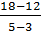### Sample Problem

Find the average rate of change between the ordered pairs  (Enter the improper fraction if necessary)

(2,18), (6,26)

(-1,14), (3,10)

(3,12), (5,18)

#### Solution

Slope1== 2

Slope2== -1

Slope3== 3

Average rate of change = (Slope1+Slope2+Slope3)/3 = (2-1+3) / 3 = 4/3